# Volume of three cuboids

Calculate the total volume of all cuboids for which the the size of the edges are in a ratio of 1:2:3, and one of the edges has a size 6 cm.

Result

V =  1506 cm3

#### Solution:Leave us a comment of example and its solution (i.e. if it is still somewhat unclear...):

Showing 0 comments:Be the first to comment!#### To solve this example are needed these knowledge from mathematics:

Tip: Our volume units converter will help you with converion of volume units.

## Next similar examples:

1. Cross-sections of a coneCone with base radius 16 cm and height 11 cm divide by parallel planes to base into three bodies. The planes divide the height of the cone into three equal parts. Determine the volume ratio of the maximum and minimum of the resulting body.
2. Cuboid and ratioCuboid has dimensions in ratio 1:2:6 and the surface area of the cuboid is 1000 dm2. Calculate the volume of the cuboid.
3. BallsPing pong balls have a diameter of approximately 4.6 cm. They are sold in boxes of 4 pieces: each box has the shape of a cuboid with a square base. The balls touch the walls of the box. Calculate what portion of the internal volume of the box is filled w
4. Carpenter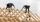From wooden block carpenter cut off a small cuboid block with half the edge length. How many percent of wood he cut off?
5. TrayWjat height reach water level in the tray shaped a cuboid, if it is 420 liters of water and bottom dimensions are 120 cm and 70 cm.
6. DiggingA pit is dug in the shape of a cuboid with dimensions 10mX8mX3m. The earth taken out is spread evenly on a rectangular plot of land with dimensions 40m X 30m. What is the increase in the level of the plot ?
7. Volume increaseHow many percent will increase in the pool 50 m, width 15m if the level rises from 1m to 150cm?
8. Juice box 2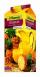Box with juice has the shape of a cuboid. Internal dimensions are 15 cm, 20 cm and 32 cm. If the box stay at the smallest base juice level reaches 4 cm below the upper base. How much internal volume of the box fills juice? How many cm below the top of the
9. Swimming poolThe pool shape of cuboid is 299 m3 full of water. Determine the dimensions of its bottom if water depth is 282 cm and one bottom dimension is 4.7 m greater than the second.
10. CuboidFind the cuboid that has the same surface area as the volume.
11. Water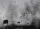In the garden with an area of 8 ares rain 40hl of water. To what heights leveled water?
12. Bottles of juice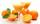How many 2-liter bottles of juice need to buy if you want to transfer juice to 50 pitchers rotary cone shape with a diameter of 24 cm and base side length of 1.5 dm.
13. GasholderThe gasholder has spherical shape with a diameter 20 m. How many m3 can hold in?
14. Theorem proveWe want to prove the sentense: If the natural number n is divisible by six, then n is divisible by three. From what assumption we started?
15. Apples in baskets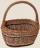Determine how many apples are in baskets when in the first basket are 4 apples, and in any other is 29 apples more than the previous, and we have eight baskets.
16. Two equationsSolve equations (use adding and subtracting of linear equations): -4x+11y=5 6x-11y=-5
17. 6 termsFind the first six terms of the sequence. a1 = 7, an = an-1 + 6